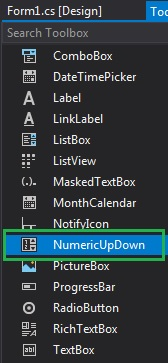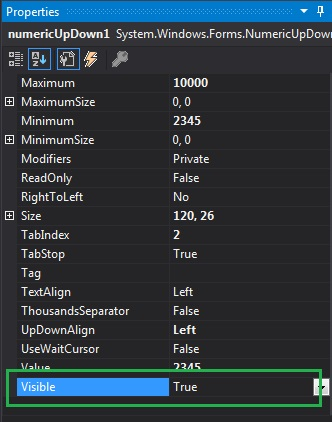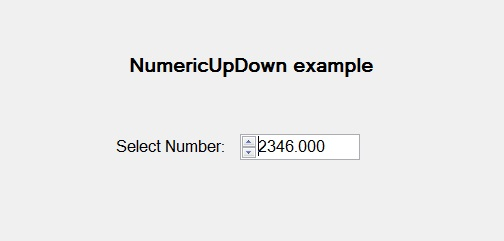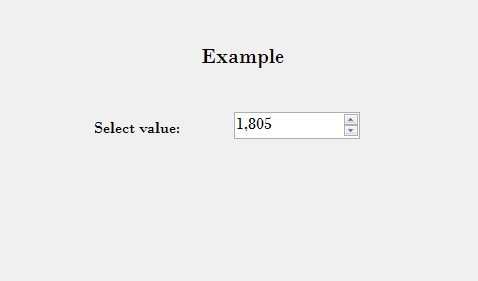# How to set the Visibility of the NumericUpDown in C#?

In Windows Forms, NumericUpDown control is used to provide a Windows spin box or an up-down control which displays the numeric values. Or in other words, NumericUpDown control provides an interface which moves using up and down arrow and holds some pre-defined numeric value. In NumericUpDown control, you can set the visibility of the up-down control on the form using Visible Property.
If the value of this property is set to true, then the NumericUpDown control display on the form. And if the value of this property is set to false, then the NumericUpDown control does not display on the form. The default value of this property is true. You can set this property in two different ways:

1. Design-Time: It is the easiest way to set the visibility of the NumericUpDown as shown in the following steps:

• Step 1: Create a windows form as shown in the below image:
Visual Studio -> File -> New -> Project -> WindowsFormApp• Step 2: Next, drag and drop the NumericUpDown control from the toolbox on the form as shown in the below image:• Step 3: After drag and drop you will go to the properties of the NumericUpDown and set the visibility of the NumericUpDown as shown in the below image:Output:2. Run-Time: It is a little bit trickier than the above method. In this method, you can set the visibility of the NumericUpDown control programmatically with the help of given syntax:

`public bool Visible { get; set; }`

The value of this property is of System.Boolean type, either true or false. The following steps show how to set the visibility of the NumericUpDown dynamically:

• Step 1: Create a NumericUpDown using the NumericUpDown() constructor is provided by the NumericUpDown class.
```// Creating a NumericUpDown
NumericUpDown n = new NumericUpDown();
```
• Step 2: After creating NumericUpDown, set the Visible property of the NumericUpDown provided by the NumericUpDown class.
```// Setting the visibility of the control
n.Visible = true;
```
• Step 3: And last add this NumericUpDown control to the form using the following statement:
```// Adding NumericUpDown control on the form
```

Example:

 `using` `System; ` `using` `System.Collections.Generic; ` `using` `System.ComponentModel; ` `using` `System.Data; ` `using` `System.Drawing; ` `using` `System.Linq; ` `using` `System.Text; ` `using` `System.Threading.Tasks; ` `using` `System.Windows.Forms; ` ` `  `namespace` `WindowsFormsApp44 { ` ` `  `public` `partial` `class` `Form1 : Form { ` ` `  `    ``public` `Form1() ` `    ``{ ` `        ``InitializeComponent(); ` `    ``} ` ` `  `    ``private` `void` `Form1_Load(``object` `sender, EventArgs e) ` `    ``{ ` `        ``// Creating and setting the ` `        ``// properties of the labels ` `        ``Label l1 = ``new` `Label(); ` `        ``l1.Location = ``new` `Point(348, 61); ` `        ``l1.Size = ``new` `Size(215, 25); ` `        ``l1.Text = ``"Example"``; ` `        ``l1.Font = ``new` `Font(``"Bodoni MT"``, 16); ` `        ``this``.Controls.Add(l1); ` ` `  `        ``Label l2 = ``new` `Label(); ` `        ``l2.Location = ``new` `Point(242, 136); ` `        ``l2.Size = ``new` `Size(103, 20); ` `        ``l2.Text = ``"Select value:"``; ` `        ``l2.Font = ``new` `Font(``"Bodoni MT"``, 12); ` `        ``this``.Controls.Add(l2); ` ` `  `        ``// Creating and setting the ` `        ``// properties of NumericUpDown ` `        ``NumericUpDown n = ``new` `NumericUpDown(); ` `        ``n.Location = ``new` `Point(386, 130); ` `        ``n.Size = ``new` `Size(126, 26); ` `        ``n.Font = ``new` `Font(``"Bodoni MT"``, 12); ` `        ``n.Minimum = 1800; ` `        ``n.Maximum = 3000; ` `        ``n.Increment = 1; ` `        ``n.ThousandsSeparator = ``true``; ` `        ``n.Visible = ``true``; ` ` `  `        ``// Adding this control ` `        ``// to the form ` `        ``this``.Controls.Add(n); ` `    ``} ` `} ` `} `

Output:Whether you're preparing for your first job interview or aiming to upskill in this ever-evolving tech landscape, GeeksforGeeks Courses are your key to success. We provide top-quality content at affordable prices, all geared towards accelerating your growth in a time-bound manner. Join the millions we've already empowered, and we're here to do the same for you. Don't miss out - check it out now!

Previous
Next